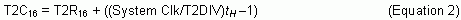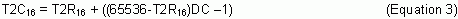# Pulse Width Modulation (PWM) with the MAXQ2000 Timer Type 2

### 要約

This application note shows how to use Timer Type 2 on a MAXQ2000 microcontroller to generate Pulse Width Modulation (PWM) waveforms. It also discusses issues that should be considered when using Timer Type 2 for PWM generation.

### Introduction

Pulse Width Modulation (PWM) is a technique in which the duty cycle of the signal conveys meaning. Specifically, the amount of time the clock signal is logic-high vs. logic-low tells the signal's receiver to do something. PWM is often used to control fan speed and DC and servo motors. With fan-speed control, the longer the high time of the pulse, the faster the fan will run. Using PWM with some external circuitry, it is even possible to implement a simple digital-to-analog converter (DAC) by charging an RC circuit of known time constant for a specific length of time. Adding a comparator circuit could create a simple analog-to-digital converter (ADC). You could even recharge a battery, which would require different external circuitry and more advanced changes.This application note explains how to use the Timer Type 2 on the MAXQ200 microcontroller to generate and control PWM.

### MAXQ2000 and Timer Type 2

The MAXQ2000 is a low-power, high-performance RISC LCD microcontroller with a large variety of peripherals and features, including three 16-bit timers. These Type 2 timers provide many functions: 8/16-bit timer/counter, autoreload, counting external pulses, capture, and compare. The Type 2 timer also provides the MAXQ2000 with PWM capability. Please see the MAXQ Users Guide (PDF) and MAXQ2000 Users Guide Supplement (PDF) for more information about the MAXQ2000 and Timer Type 2.

### PWM Signal Generation

The MAXQ2000's Timer Type 2 utilizes autoreload with compare to generate the PWM signal. Every time the timer overflows or has a compare value match, it toggles the logic state of the output pins T2P and T2PB. Figure 1 shows a basic PWM waveform in which the base period (1/fB) corresponds to the timer's overflow. The timer and reload register should be set to a value that will overflow and reload every 1/fB seconds. Use Equation 1 to determine this value:Where T2R16 is the initial value of Timer Type 2 as well as the reload value. System Clk may be either the actual system clock, i.e., 16MHz, or optionally the 32kHz clock. If the base frequency, fB, is significantly smaller than the system frequency, T2DIV can be used to divide the input clock down to a more reasonable level.

The high time of the PWM waveform, tH, corresponds to a match of the timer compare register. The compare register should be set to a value that will match tH after every reload. Use Equation 2 for this. It is important to remember that tH must be less than the base period. Note, also, that compare output toggle occurs on the next timer tick after a match, which explains the -1 in the following equation. If the high time and the base period were the same, the output waveform would only toggle one time on overflow, resulting in a half-speed wave that will cause problems.In some applications tH may be described by a percentage or duty cycle (DC). In this case, use the following Equation 3, where a DC of 50% (half high, half low) is represented as 0.50.Figure 1. PWM waveform.

### Considerations

Some PWM applications require changing either the base frequency of the waveform or modifing the high time. It is important to stop the timer using TR2 before making these changes. Otherwise, the PWM waveform can invert because the timer toggles the output an additional time. Stopping and restarting the timer guarantees a consistent waveform.

Some hardware is sensitive to the PWM glitch resulting from stopping and restarting the PWM waveform as described above. If glitch-free PWM is required, more care must be taken when updating timer registers. One scheme uses the overflow and compare flags as signals to update. When the compare flag/interrupt flag is set, update the reload register. When the overflow flag is set, update the compare register. This scheme allows changes to occur in a predictable manner. Note that problems can occur with this scheme near boundaries. Specifically, if timer input is an undivided system clock, attempting to update the compare register to one count more than the reload can cause unexpected behavior.

Because of the output's toggling behavior, it is important to consider the initial state of the pin. The initial state of the output is controlled by T2POL; if set to 0, it inverts the output signal. This behavior can be seen in the dual 8-bit timer example in the Appendix below.

The MAXQ2000 and Timer Type 2 support many options required for specialized applications. It is possible to gate the PWM signal on the secondary output pin by using the primary output pin as a gating input. This effectively stops the timer tick and will stop the PWM signal. Other specialized modes include single-shot and gated single-shot.

Timer Type 2 also supports PWM signal generation in three 8-bit modes. These modes allow the timer to be used for multiple tasks when the greater precision of the 16-bit timer is not required. The dual 8-bit mode allows two unique PWM signals to be generated by the timer, while the other 8-bit modes only support PWM output on the secondary pin, T2PB. To configure the timers in the 8-bit modes, use Equations 1 and 2 for reload and compare values, except substitute 256 for 65536.

The system clock frequency affects the accuracy of the output waveform. A 16MHz clock, for example, divides by 8 much more evenly than a 14.7456MHhz clock. Many fast timer ticks allow better accuracy than two large slow ones. The 16-bit timer is more accurate than the 8-bit timer for this reason.

### Example Code

Appendix A contains code samples for setting up PWM on Timer 0 of a MAXQ2000 in both 16-bit and dual 8-bit modes.

### Appendix A. Code Samples

```; 16 bit PWM example code
; MAXQ2000 has 3 Type 2 Timer/Counters with 2 output pins each
; System Clock is 16Mhz
; We will use timer 0

\$include (maxQ2000.inc)

; Some basic initialization.  Set Accumulator Controls to normal.
move  APC, #0           ; no accumulator-modulo behavior
move  AP,  #0           ; select accumulator 0

move T2CFG0, #00000000b ; Configure the Timer
; T2CI = 0   - Use System clock
; TDIV = 000 - Use divide by 1
; T2MD = 0   - 16 bit
; CCF  = 00  - Compare/Reload mode
; C/T2 = 0   - Timer mode

move T2CNA0, #01100000b ; Set up Timer Control
; ET2    = 0  - No interrupts
; T2OE0  = 1  - Utilize Primary output pin
; T2POL0 = 1  - Initial output high
; TR2L   = 0  - Don't care in 16 bit mode
; TR2    = 0  - Don't run yet
; CPRL2  = 0  - Don't care in compare mode
; SS2    = 0  - No Single Shot
; G2EN   = 0  - No Clock Gating

move T2V0, #63936       ; Set initial timer count to generate
; 10Khz base frequency PWM wave

move T2R0, #63936       ; Set Reload to same value

;	move T2C0, #64735       ; 50% Duty
;	move T2C0, #64095       ; 10% Duty
move T2C0, #65135       ; 75% Duty

move acc, T2CNA0
or   #00001000b         ; Start the Timer
move T2CNA0, acc

jump \$
end

; Dual 8-bit PWM example code
; Timers will output inverse wave forms
; MAXQ2000 has 3 Type 2 Timer/Counters with 2 output pins each
; System Clock is 16Mhz
; We will use timer 0

\$include (maxQ2000.inc);

; Some basic initialization.  Set Accumulator Controls to normal.
move  APC, #0           ; no accumulator-modulo behavior
move  AP,  #0           ; select accumulator 0

move T2CFG0, #00111000b ; Configure the Timer
; T2CI = 0   - Use System clock
; TDIV = 011 - Use divide by 8
; T2MD = 1   - 8 bit
; CCF  = 00  - Compare/Reload mode
; C/T2 = 0   - Timer mode

move T2CNA0, #01000000b ; Set up Timer Control
; ET2    = 0  - No interrupts
; T2OE0  = 1  - Utilize Primary output pin
; T2POL0 = 0  - Initial output low
; TR2L   = 0  - Don't run lower yet
; TR2    = 0  - Don't run upper yet
; CPRL2  = 0  - Don't care in compare mode
; SS2    = 0  - No Single Shot
; G2EN   = 0  - No Clock Gating

move T2CNB0, #01100000b ; Set up Timer Control B
; ETl2   = 0  - No interrupts
; T2OE1  = 1  - Utilize Primary output pin
; T2POL1 = 1  - Initial output high
; reserved
; 3:0 - interrupt flags

move T2V0, #56          ; Set initial timers count to generate
move T2H0, #56		; 10Khz base frequency PWM wave

move T2R0, #56	      ; Set Reload to same value
move T2RH0, #56

move T2C0, #95          ; Set high time for 20us
move T2CH0, #95

move acc, T2CNA0
or   #00011000b         ; Start the Timer
move T2CNA0, acc

jump \$
end
```